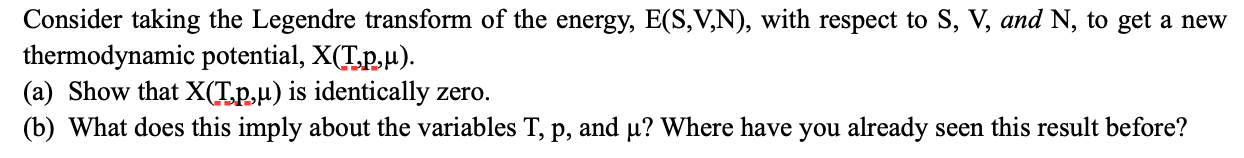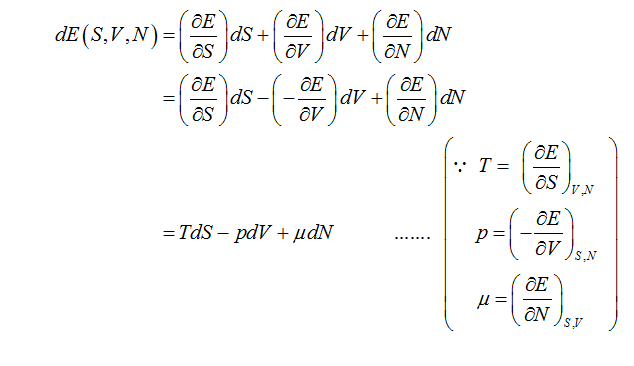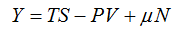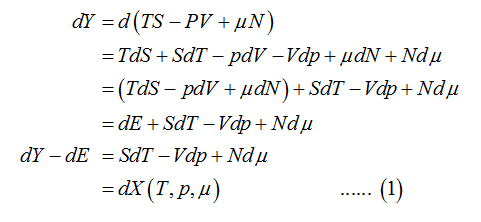# Consider taking the Legendre transform of the energy, E(S,V,N), with respect to S, V, and N, to get a newthermodynamic potential, X(T.p,u).(a) Show that X(T.p,µ) is identically zero.(b) What does this imply about the variables T, p, and µ? Where have you already seen this result before?

Question
1 views

It's a thermodynamics question.help_outlineImage TranscriptioncloseConsider taking the Legendre transform of the energy, E(S,V,N), with respect to S, V, and N, to get a new thermodynamic potential, X(T.p,u). (a) Show that X(T.p,µ) is identically zero. (b) What does this imply about the variables T, p, and µ? Where have you already seen this result before? fullscreen
check_circle

star
star
star
star
star
1 Rating
Step 1

Write the expression for the total differential of the energy E given as a function of entropy S, volume V, and number of particles N.Step 2

Here T is the temperature, p is the pressure and μ is the chemical potential.

In the above expression, the conjugate pair of variables are (T, S), (p, V) and (μ, N).

To obtain the function X (T, p, μ) through Legendre transformation, consider an arbitrary function Y that assumes the following form.Step 3

Take the total differential of above function and simplify the expression....

### Want to see the full answer?

See Solution

#### Want to see this answer and more?

Solutions are written by subject experts who are available 24/7. Questions are typically answered within 1 hour.*

See Solution
*Response times may vary by subject and question.
Tagged in

### Science